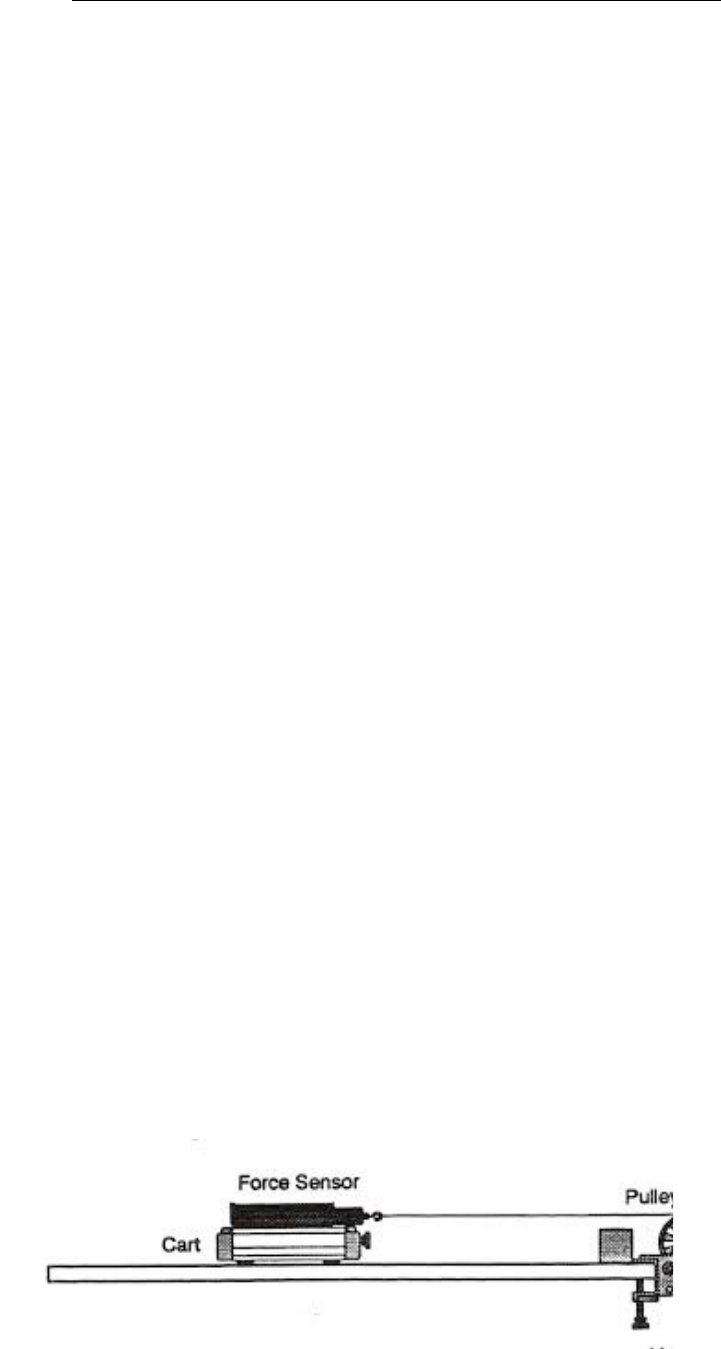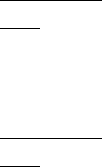Textbook Notes (270,000)
CA (160,000)
McMaster (10,000)
BIOLOGY (700)
Chiu (2)
Chapter

Exploring the Force.docx

Department
Biology
Course Code
BIOLOGY 3MM3
Professor
Chiu

This preview shows pages 1-2. to view the full 7 pages of the document.Exploring the Force, Mass and Acceleration Relationship
Purpose:
The purpose of this experiment is to determine the relationship between force
and acceleration and mass and acceleration in accordance with Newton’s 2nd
Law of Motion.
Hypothesis:
According to Newton’s second law of motion Fnet  a if mass remains constant,
where Fnet represents the force acting on the cart (N) and a represents
acceleration of the cart in (m/s2). Also a  1/m when force remains constant,
where m represents the mass. As a result the acceleration versus force graph will
yield a positive sloping linear graph, where as the acceleration versus 1/mass
graph will yield an exponential function.
Materials:
Cart, pulley, varying steel masses, acceleration device
Safety Precautions:
Have group member keep cart on the desk to prevent it from falling on
legs and toes
Make sure device is plugged in and wires are kept low to the ground to
avoid tripping and falling.
Ensure steel masses are properly on table to avoid falling on toes
Procedure:
1. Placed hanging mass on pulley and ensured accelerator device was
working properly.
2. Released cart and values were recorded.
3. Repeated steps 1 and 2 for each of the three trials.
4. Began experiment two by removing five masses from the hanging mass
system and recorded values of acceleration.
5. Repeated step 4, but this time added two masses to the cart.
6. Values were then observed and recorded for all three trials.
Set up:

Only pages 1-2 are available for preview. Some parts have been intentionally blurred.Observations: Observations of acceleration of toy cart moving on horizontal
surface
Cart
Mass
(Kg)
Hangin
g Mass
(Kg)
Mass
of
Syste
m (Kg)
Force
(N)
Accelerati
on 1 (m/s2)
Accelerati
on 2 (m/s2)
Average
Accelerati
on
Experime
nt 1
0.5 0.18 0.68 1.76 2.7 2.37 2.54
0.53 0.15 0.68 1.47 2.41 2.14 2.28
0.57 0.11 0.68 1.08 1.66 1.71 1.69
Experime
nt 2
0.52 0.11 0.63 1.08 1.38 1.42 1.40
0.54 0.11 0.65 1.08 1.37 1.31 1.34
0.57 0.11 0.68 1.08 1.32 1.09 1.21
Experiment 1 – The Effect of Force on Acceleration
Variables:
Independent Variable: Force
Dependent Variable: Acceleration
Constants: Mass of System
**Black line represents line of best fit**
Calculations: